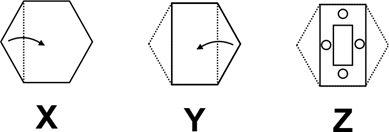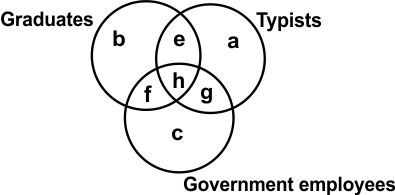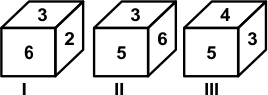# CREST Reasoning Olympiad Class 10 Sample Papers

REGISTER NOW

## Syllabus:

Section 1: Series Completion, Analogy and Classification, Coding - Decoding, Blood Relations, Sequential Output Tracing , Direction Sense Test, Logical Venn Diagrams, Alphabet Test and Logical, Alpha-Numeric, Number, Mathematical Operations, Inserting the Missing Characters, Analytical Reasoning, Mirror Images and Water Images, Spotting Out The Embedded, Figure Matrix, Paper Folding and Paper Cutting, Grouping of Identical Figures, Cubes and Dice, Dot Situation, Construction of Squares and Triangles, Figure Formation and Analysis.

Achievers Section: Higher Order Thinking Questions - Syllabus as per Section 1

 Q.1 If '+' means '-', '÷' means '+', '-' means '×' and '×' means '÷', then which of the following equation is correct?
 Q.2 Amongst four friends Sakshi, Bhumika, Gitika and Chetna, Bhumika is taller than Chetna but not taller than Sakshi, Gitika's height is shorter than that of Sakshi. Which of the following is not possible?
 Q.3 In the following question a piece of paper is folded in the manner shown in the question figure(s). Select the figure out of the answer choices showing the unfolded pattern after the punches (cut).Q.4 The figure given below is made of three circles intersecting one another. These circles represent graduates, typists and government employees. The intersecting regions have been denoted by a, b, c, e, f, g and h, respectively. Study the diagram carefully and answer the question that follows. Which of the following letters represents the typists are only graduates?Q.5 Choose the missing term in the given series. BC, EFG, IJKL, ?, TUVWXY
 Q.6 Pointing towards photograph, Rohan said, "His sister is the mother of Rohit who is the son of my brother." How Rohan is related to Rohit?
 Q.7 Study the following information carefully to answer the question.I. 'A \$ B' means 'A is the mother of B'.II. 'A # B‘ means 'A is the father of B'.III. 'A @ B' means 'A is the husband of B'. IV. 'A % B' means 'A is the daughter of B'. If F@D%K#H, then how is F related to H?
 Q.8 Study the given relationship and complete the following analogies from the two sets of options given. Square is to (i), as Circle is to (ii)(i) [A] Rectangle [B] Cube [C] Cuboid [O] Triangle(ii) [W] Ellipse [X] Ellipsoid [Y] Sphere [X] Line
 Q.9 Three positions of a dice are given below. Identify the number on the face opposite to 6.Q.10 In this question, relationship between different elements is shown in the statements. The statements are followed by two conclusions. Statements: A > B, B = H, H > GConclusions: I. A > G, II. A > H

Sample PDF of CREST Reasoning Olympiad for Class 10: